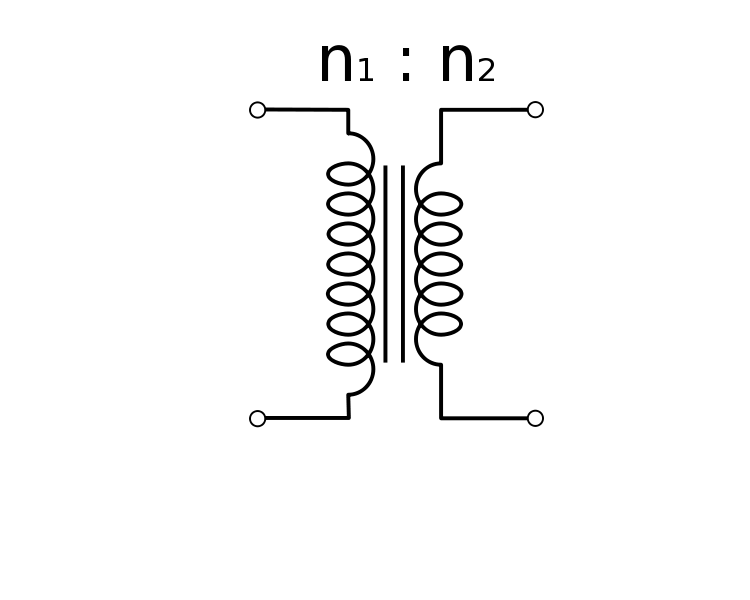Transformer is a electrical device and it is used to convert low alternating voltage to high alternating voltage and vice versa. Step up transformer converts low voltage to high voltage and Step down transformer converts high voltage to low voltage.

A transformer works on the principle of electro magnetic induction. A transformer consists of primary and secondary coils insulated from each other. Both coils are wounded around soft iron core. Primary coil is wounded around one end and Secondary coil is wounded around another opposite end. Alternating Voltage (input) is applied across primary coil and alternating current flows through the primary coil. The current in primary coil creates varying magnetic flux in the same coil, which in turn produces varying magnetic field in the secondary coil. Hence, Electro motive force (EMF) is induced across the secondary coil.

The terms encountered in transformer circuit are induced EMF in primary and secondary coils (Ep &Es), current through both coils (Ip & Is) and number of turns in coils ( Np & Ns).All these terms can be combined in one equation as follows,

##### Es/Ep = Ns/Np = Ip/Is =k

where k is transformer ratio. For step up transformer, k>1 and for step down transformer, k<1.

When step up transformer functioning, where do it gain to increase voltage from? Conservation of energy must be noted here. By decreasing current, Step up transformer increases alternating voltage. It can be clearly noted from power equation.

##### Ep Ip (input power) = Es Is (output power)

There will be no change in output power as compared to input power, but voltage (Es) and current (Is) are adjusted according step up and step down transformer.